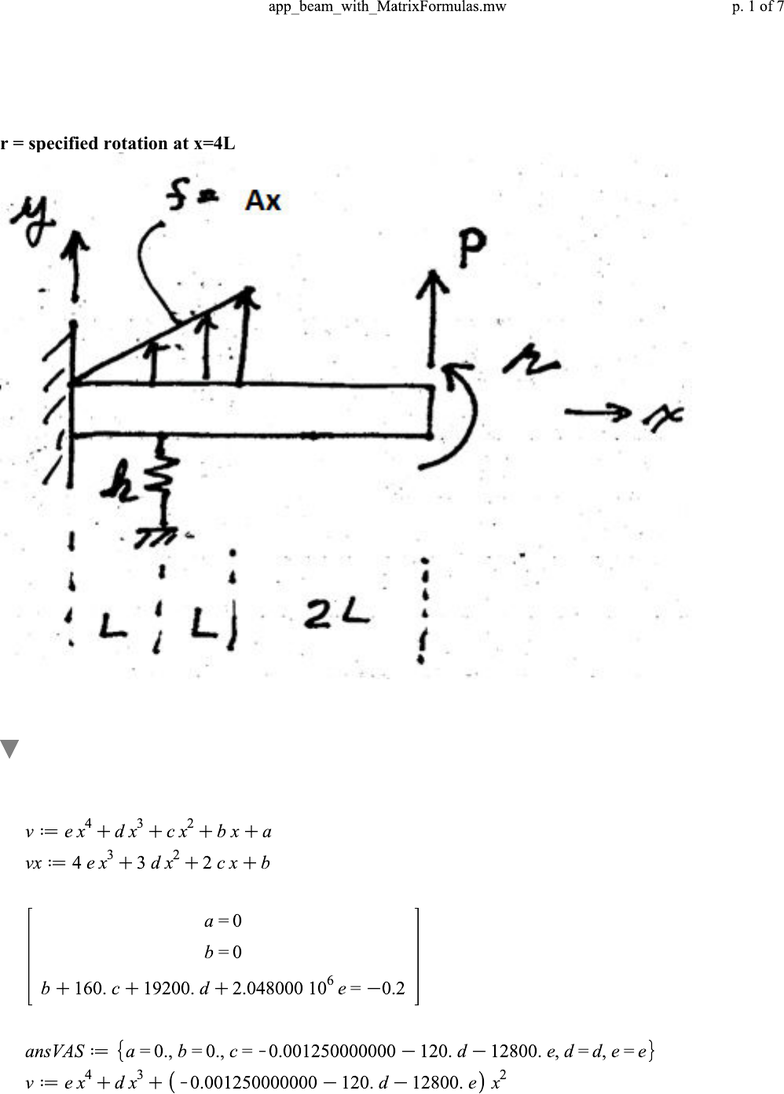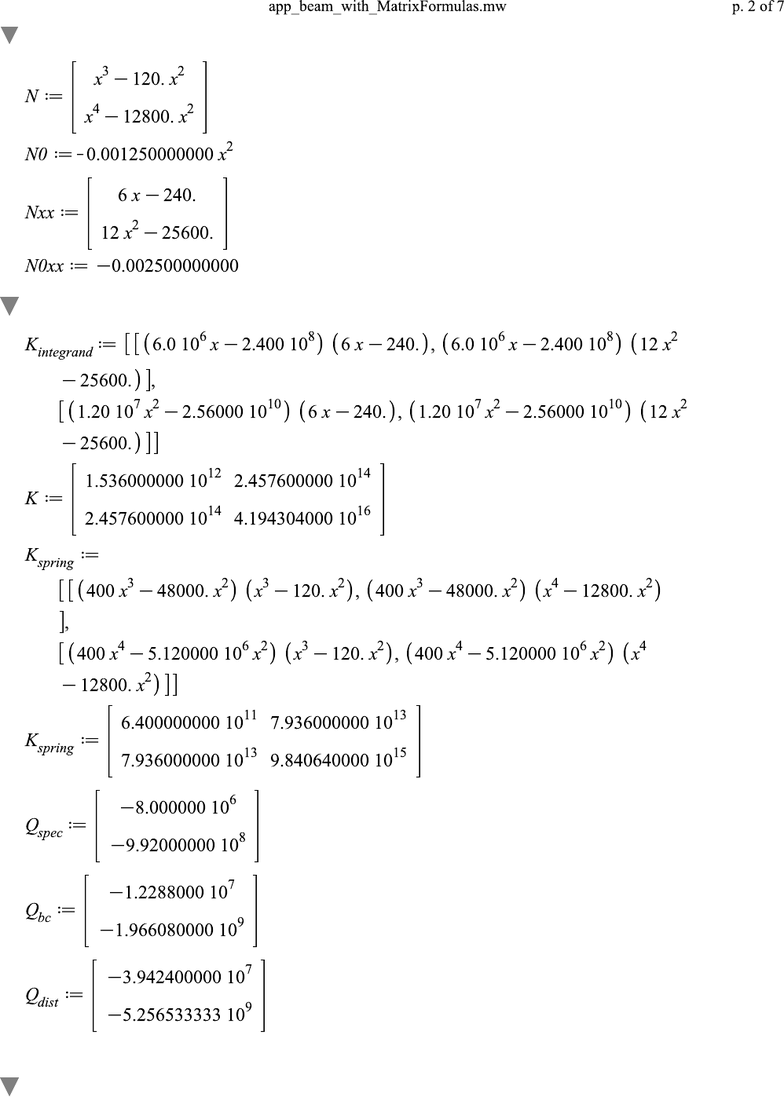Study Guides (390,000)
US (220,000)
TAMU (3,000)
AERO (10)
Final

# AERO 306 Lecture 8: app_beam_with_MatrixFormulas_codeHiddenExam

Department
Aerospace Engineering
Course Code
AERO 306
Professor
John Whitcomb
Study Guide
Final

This preview shows pages 1-2. to view the full 7 pages of the document.(1.1)(1.1)
Analysis of a Beam with a Specified Rotation
Goal: 2-term approximate solution using matrix formulas
I have modified the code to make the spring located a distance "L__spring" from the left.
r = specified rotation at x=4L
You can simplify this problem by simply setting some parameters like k or P to zero at the beginning. If
you set r=0, then you have the zero rotation condition, which is also simpler.
Get Valid assumed solution
There are three kinematic constraints, so we need to start with 5 terms.
Comment out the next line except when generating results for pasting into the Word file.
The equations to be solved to impose the kinematic constraints are
After the constraints are imposed, we have the following valid assumed solution

Only pages 1-2 are available for preview. Some parts have been intentionally blurred.(3.2)(3.2)
(3.4)(3.4)
(2.1)(2.1)
(3.3)(3.3)
(3.5)(3.5)
(3.1)(3.1)
(2.2)(2.2)
Pick out the interpolation functions
Calculate the stiffness matrix and load terms
Solve